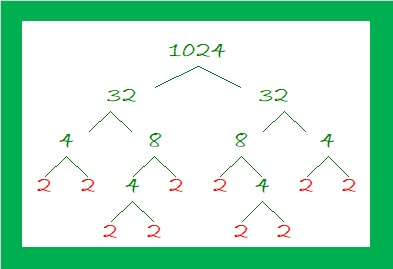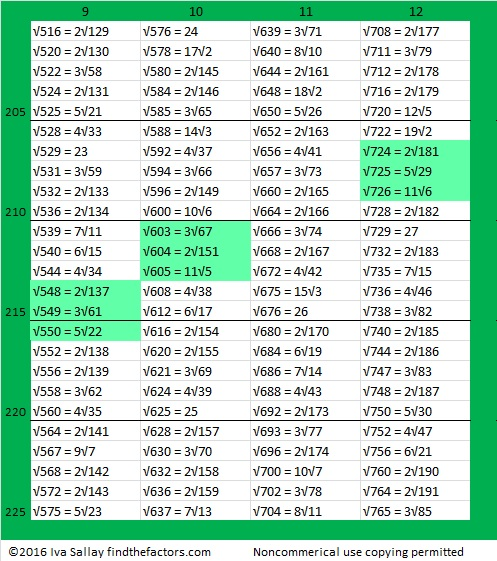# What’s Special About √1024?

What’s special about √1024? Is it because it and several counting numbers after it have square roots that can be simplified?Perhaps.

Maybe it is interesting just because √1024 = 32, a whole number. The 5th root of 1024 = 4 and the 10th root of 1024 = 2, both whole numbers as well.

Those equations are true because 32² = 1024, 4⁵ = 1024, and 2¹⁰ = 1024.

Or perhaps 1024 is special because it is the smallest number that is a 10th power. (It is 2¹⁰.) The square root of a perfect 10th power is always a perfect 5th power. (32 = 2⁵ and is the smallest number that is a 5th power.)

1024 is also the smallest number with exactly 11 factors.

It is the smallest number whose factor tree has at least 10 leaves that are prime numbers. (They are the red leaves on the factor tree shown below.) It is possible to draw several other factor trees for 1024, but they will all have the number 2 appearing ten times.What’s more, I noticed something about 1024 and some other multiples of 256: Where do multiples of 256 fall on the list of square roots that can be simplified?

• 256 × 1 = 256 and 256 is the 100th number on this list of numbers whose square roots can be simplified.• 256 × 2 = 512. When we add the next 100 square roots that can be simplified, 512 is the 199th number on the list.• Here are the third 100 square roots that can be simplified:• 256 × 3 = 768 didn’t quite make that list because it is the 301st number. Indeed, it is the first number on this list of the fourth 100 numbers whose square roots can be simplified.• 256 × 4 = 1024. That will be the first number on the 5th 100 square roots list!

It is interesting that those multiples of 256 have the 100th, the 199th, the 301st, and the 401st positions on the list. That is so close to the 100th, 200th, 300th, and 400th positions.

In case you couldn’t figure it out, the highlighted square roots are three or more consecutive numbers that appear on the list.

1024 is interesting for many other reasons. Here are a few of them:

(4-2)¹⁰ = 1024, making 1024 the 16th Friedman number.

I like to remember that 2¹⁰ = 1024, which is just a little bit more than a thousand. Likewise 2²⁰ = 1,048,576 which is about a million. 2³⁰ is about a billion, and 2⁴⁰ is about a trillion.

*******
As stated in the comments, Paula Beardell Krieg shared a related post with me. It takes exactly 1024 Legos to build this fabulous pyramidal fractal:

1024 has so many factors that are divisible by 4 that it is a leg in NINE Pythagorean triples:
768-1024-1280 which is (3-4-5) times 256
1024-1920-2176 which is (8-15-17) times 128
1024-4032-4160 which is (16-63-65) times 64
1024-8160-8224 which is (32-255-257) times 32
1024-16368-16400 which is 16 times (64-1023-1025)
1024-32760-32776 which is 8 times (128-4095-4097)
1024-65532-65540 which is 4 times (256-16383-16385)
1024-131070-131074 which is 2 times (512-65535-65537),
and primitive 1024-262143-262145

Some of those triples can also be found because 1024 is the difference of two squares four different ways:
257² – 255² = 1024
130² – 126² = 1024
68² – 60² = 1024
40² – 24² = 1024
To find out which difference of two squares go with which triples, add the squares instead of subtracting and you’ll get the hypotenuse of the triple.
******

1024 looks interesting in some other bases:
It’s 1000000000 in BASE 2,
100000 in BASE 4,
2000 in BASE 8,
1357 in BASE 9,
484 in BASE 15,
400 in BASE 16,
169 in BASE 29,
144 in BASE 30,
121 in BASE 31, and
100 in BASE 32

• 1024 is a composite number.
• Prime factorization: 1024 = 2 × 2 × 2 × 2 × 2 × 2 × 2 × 2 × 2 × 2, which can be written 1024 = 2¹⁰
• The exponent in the prime factorization is 10. Adding one we get (10 + 1) = 11. Therefore 1024 has exactly 11 factors.
• Factors of 1024: 1, 2, 4, 8, 16, 32, 64, 128, 256, 512, 1024
• Factor pairs: 1024 = 1 × 1024, 2 × 512, 4 × 256, 8 × 128, 16 × 64, or 32 × 32,
• 1024 is a perfect square. √1024 = 32. It is also a perfect 5th power, and a perfect 10th power.•ivasallay